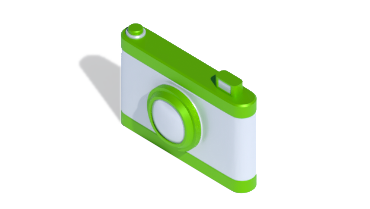Delivery by Thursday if you order in nextTalk to Swiftpak 0118 916 7320

## Product Options

Code Name Units
Per Pack

Quantity

Total
Price
TO08HSK
1
1 total units
TO12HEATE
1
1 total units
TO12HEATT1
1 total units
TO12HSK
1
1 total units
TO16HEATE
1
1 total units
TO16HSK
1
1 total units
TOHSCBH
1
1 total units
• Code TO08HSK
• Name
Quantity
1 total units

Total Price

• Code TO12HEATE
• Name
Quantity
1 total units

Total Price• Code TO12HEATT
• Name
Quantity
1 total units

Total Price

• Code TO12HSK
• Name
Quantity
1 total units

Total Price

• Code TO16HEATE
• Name
Quantity
1 total units

Total Price

• Code TO16HSK
• Name
Quantity
1 total units

Total Price

• Code TOHSCBH
• Name
Quantity
1 total units

Total Price